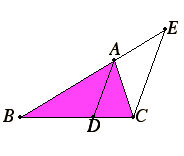# Proposition 3

If an angle of a triangle is bisected by a straight line cutting the base, then the segments of the base have the same ratio as the remaining sides of the triangle; and, if segments of the base have the same ratio as the remaining sides of the triangle, then the straight line joining the vertex to the point of section bisects the angle of the triangle.

Let ABC be a triangle, and let the angle BAC be bisected by the straight line AD.

I say that DB is to DC as AB is to AC.I.31

Draw CE through C parallel to DA, and carry AB through to meet it at E.

I.29

Then, since the straight line AC falls upon the parallels AD and EC, the angle ACE equals the angle CAD.

But the angle CAD equals the angle BAD by hypothesis, therefore the angle BAD also equals the angle ACE.

I.29

Again, since the straight line BAE falls upon the parallels AD and EC, the exterior angle BAD equals the interior angle AEC.

I.6

But the angle ACE was also proved equal to the angle BAD, therefore the angle ACE also equals the angle AEC, so that the side AE also equals the side AC.

VI.2

And, since AD is parallel to EC, one of the sides of the triangle BCE, therefore, proportionally DB is to DC as AB is to AE.

V.7

But AE equals AC, therefore DB is to DC as AB is to AC.

Next, let DB be to DC as AB is to AC. Join AD.

I say that the straight line AD bisects the angle BAC.

With the same construction, since DB is to DC as AB is to AC, and also DB is to DC as AB is to AE, for AD is parallel to EC, one of the sides of the triangle BCE, therefore also AB is to AC as AB is to AE.

Therefore AC equals AE, so that the angle AEC also equals the angle ACE.

I.29

But the angle AEC equals the exterior angle BAD, and the angle ACE equals the alternate angle CAD, therefore the angle BAD also equals the angle CAD.

Therefore the straight line AD bisects the angle BAC.

Therefore, if an angle of a triangle is bisected by a straight line cutting the base, then the segments of the base have the same ratio as the remaining sides of the triangle; and, if segments of the base have the same ratio as the remaining sides of the triangle, then the straight line joining the vertex to the point of section bisects the angle of the triangle.

Q.E.D.

## Guide

This proposition characterizes an angle bisector of an angle in a triangle as the line that partitions the base into parts proportional to the adjacent sides. The second part of the statement of the proposition is the converse of the first part of the statement. The proof relies on basic properties of triangles and parallel lines developed in Book I along with the result of the previous proposition VI.2.

#### Use of this proposition

This proposition is not used in the remainder of the Elements.